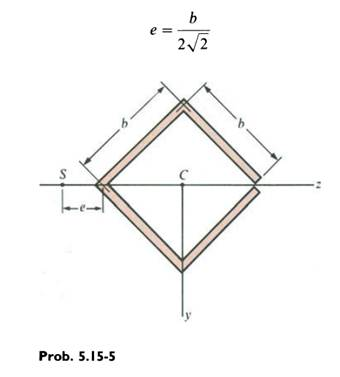### Create an Account

Home / Questions / A cross section of a slit square tube of constant thickness is shown in the figure Derive ...

# A cross section of a slit square tube of constant thickness is shown in the figure Derive the following formula for the distance e from the corner of the cross section to the shear center

A cross section of a slit square tube of constant thickness is shown in the figure. Derive the following formula for the distance e from the corner of the cross section to the shear center S:Jun 29 2020 View more View LessSubscribe To Get Solution